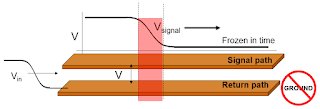## You need to test, we're here to help.

You need to test, we're here to help.

## 26 February 2018

### Transmission Lines (Part III): Essential PrinciplesFigure 1: All interconnects are transmission lines with a signal path and a return path (not ground)
Now that we've covered some of the principles and assumptions that underlie transmission lines in two prior posts, we can now directly address the topic with some essential principles that you need to understand.

The first principle is that all interconnects are transmission lines, with no exceptions (Figure 1). When we look at, say, a printed-circuit board, we see surface traces that carry signals. But we're only seeing half of the transmission line, because associated with each of these signal traces is a return path.

Every transmission line requires two conductors with some length: a signal path and a return path. An unhelpful habit that we need to unlearn is referring to that return path as "ground." Using the term "return path" is a much more helpful way of thinking about the other conductor in the transmission line and will reinforce better intuition for the purpose of problem solving.

So if we can see the signal paths on a PCB in the form of the surface traces, what comprises the return paths? It's that thing we used to call "ground": the plane beneath the surface traces. It's covered by the solder mask, but whenever we think about the surface traces, we should be mindful of that other half of the transmission line.

Our second essential principle of transmission lines is that signals are dynamic. When we launch a signal onto a transmission line, what constitutes that signal is a voltage difference between the signal and return paths (voltage V in Figure 1). And when we launch that voltage difference, it will propagate, and there's absolutely nothing we can do about that. That voltage difference will travel the length of the transmission line at some rate of speed.

How fast will that voltage difference travel? It travels at the speed of light in the dielectric material, which is expressed as:

In FR-4 PCB material, the dielectric constant is about 4, so in PCBs, the speed of signals is:

Meanwhile, with a dielectric constant of about 2.2, the speed of signals in coaxial cable is:

So for a 1' length of coax, the time delay for a signal from end to end is about 1.5 ns.Figure 2: Signals see an instantaneous impedance as they traverse a transmission line
Principle #3: Signals see an instantaneous impedance. In Figure 2, imagine that some fraction of a nanosecond after we launched a 1-V signal onto a transmission line, we were able to stop time. In the moment that the signal launched, it saw an impedance. We can think of that edge as a ripple rolling down the transmission line. To the left of the red-shaded section, there is a potential of 1 V between the signal and return paths. To the right of that section, there's no voltage because the signal has not gotten there yet.

That signal sees that impedance as it rolls along, and let's remember the fundamental definition of impedance: the ratio of voltage over current. So as our signal moves along the transmission line, it charges each successive region of the line to 1 V. The edge of our signal has a spatial extent at which there is a dV/dt and dI/dt. Every step the signal takes, it leaves the same amount of charge in its wake. That successive amount of charge in each unit of time is a current. If the transmission line's cross section is uniform along its length, that instantaneous impedance will be constant as the signal propagates through.

In the case of a coaxial cable, the instantaneous impedance a launched signal sees is 50 Ω. With its uniform cross section, each successive region of that coaxial cable will present that instantaneous impedance to our signal, and it will take the same amount of charge for each successive region. Thus, there is one value of instantaneous impedance that characterizes a coaxial transmission line, and we call that its characteristic impedance.

We'll continue with essential principles of transmission lines in a subsequent post.

Previous posts in this series:

Transmission Lines (Part I): Introduction
Transmission Lines (Part II): More on Bandwidth vs. Rise Time